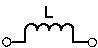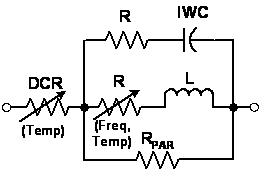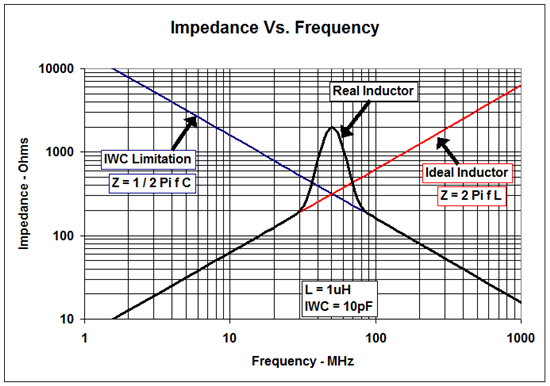# When to use an RF choke vs an inductor

Most engineers are familiar with inductors as they are a key element in many frequency selective circuits.  One application for inductors though that you may not be quite as familiar with is as an RF choke.

An RF choke is an inductor, however the frequency selectivity is simply to pass DC and block everything else.

In this application the usual figures of merit for inductors may not adequately capture the actual desired performance of the inductor.Ideal Model

In many applications inductors are used to pass or block signals.  When used as an RF choke, an inductor should only pass DC, everything else is blocked.  Unfortunately, blocking very low frequencies requires a very large inductor.   How do we choose an ideal component?

When designing a filter the Q, or quality factor, of an inductor is very important and the goal is a high Q component.  When selecting an RF choke, a high Q inductor isn’t always the best choice.  High Q means low loss, but for an RF choke higher loss means better isolation.  This means for a given inductance, a lower Q choke has more parasitic resistance than a high Q choke.   Another consideration is that Q is frequency dependent, often heavily so.Better Model

When choosing an RF choke, a high value of inductance is usually desired. For example, in the LMH6521 datasheet we recommend an inductor with at least 1uH of inductance. This allows low frequency signals to pass with minimal attenuation.  In the LMH6515 datasheet, Figure 42 shows the impact of different inductor values on the frequency response.    A high value inductor usually has a fairly high level of parasitic capacitance and consequently a low self-resonant frequency.   At this point it is important to carefully read the datasheet.  In theory, an inductor behaves as a capacitor when used above the self-resonant frequency (see graph below).  This may be the case for high Q inductors, but many RF chokes have thin wires to pack a given inductance into a small space (see the Best Model below).  Due to the impact of skin effect these RF chokes often do not have enough capacitance to counteract the high frequency resistance of the wire.  This means that they may be useful for a wider frequency range than the datasheet indicates, sometimes up to several orders of magnitude higher frequency than the self-resonant frequency shown in the datasheet.Best ModelFor a step by step approach to selecting an RF choke use these steps:

1.  Make sure the DC current capability matches the required bias current.
2. Select a sufficiently high inductance value. (Z = 2 * pi * f * L) Select an impedance (Z) that is much larger than the load resistance.
3. Decide the maximum allowable DC resistance so that the voltage drop on the inductor is small compared to the voltage swing of the amplifier stage.  A resistance of between 1 and 10 Ohms is usually OK.
4. Select a small package size.
5. Look for a low Q inductor (High L and small physical size usually means Low Q).
6. Make sure the core material is useable in the frequency range of interest.

If you like what you read here, check out my other blog posts for additional info.

Learn about TI’s entire portfolio of amplifier ICs and find technical resources.

• Hello Stevan,

You would want to consider the application.  Ferrite beads are usually used to block lower frequencies than RF chokes. For example, ferrite beads are used on AC power lines and for switching power supplies.  The core of the ferrite bead usually has very high permability at low frequencies, but at RF may have not much permability left. RF inductors will either use an RF ferrite or simply a lot of windings on an air core.

Regards,

Loren

• Great article, thanks. When would I use a ferrite bead vs inductor?